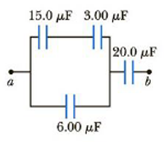Chapter 16, Problem 48P

Chapter
Section
Textbook Problem

Four capacitors are connected as shown in Figure P16.48.(a) Find the equivalent capacitance between points a and b.(b) Calculate the charge on each capacitor, taking ΔVab = 15.0 V.Figure P16.48

(a)

To determine
The equivalent capacitance.

Explanation

The capacitors 15.0μF and 3.00μF are connected in series combination. The equivalent capacitance is,

Cs=C15.0μFC3.00μFC15.0μF+C3.00μF

The capacitors Cs and 6.00μF are connected in parallel combination. The equivalent capacitance is,

Cp=Cs+(6.00μF)

The capacitance Cp and 20.0μF are connected in series combination. The total equivalent capacitance is,

Ceq=(C15.0μFC3.00μFC15.0μF+C3.00μF+C6.00μF)(C20.0μF)(C15.0μFC3.00μFC15.0μF+C3.00μF+C6.00μF)+(C20

(b)

To determine
The charge on each capacitor.

Still sussing out bartleby?

Check out a sample textbook solution.

See a sample solution

The Solution to Your Study Problems

Bartleby provides explanations to thousands of textbook problems written by our experts, many with advanced degrees!

Get Started